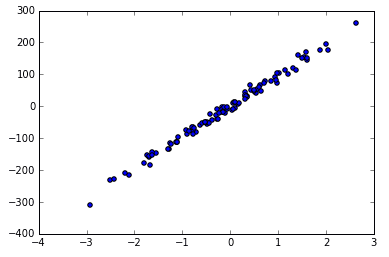半监督学习

参考

1. 小海星机器学习讲义
2. https://en.wikipedia.org/wiki/Semi-supervised_learning
3. https://en.wikipedia.org/wiki/Weak_supervision

ML 的定义

An approach to achieve artificial intelligence through systems that can learn from experience to find patterns in that data

ML teaches a computer to recognise patterns by examples, rather than programming it with specific rules.feature机器学习的分类

无监督的学习例子

线性回归（Linear Regression）聚类KNN神经网络fully-connected 神经网络卷积神经网络（CNN）

CNN 可以用来处理图片数据，以及可以表示为图片的数据。要确定一组数据可不可以表示为图片，可以尝试交换任意两行，或者任意两列，如果交换后对数据没有影响，那么就不可以被认为是图片数据。更多细节参看这个 Video

算法的分类

RegressionInstance Based

Instance Based 是一个决策方法。通过相似度计算找出最接近的实例。比如KNNDecision TreeBayesianclusteringassociation rulesArtificial Neural Network深度学习降维 (Dimensionality Reduction)sklearn 入门笔记

什么是机器学习？

sklearn 给了一个定义

In general, a learning problem considers a set of n samples of data and then tries to predict properties of unknown data. If each sample is more than a single number and, for instance, a multi-dimensional entry (aka multivariate data), it is said to have several attributes or features.

要解决的问题

1. Classification 分类，也就是离散的
2. Regression 回归，也就是连续的
3. Clustering 聚类
4. Dimensionality reduction 数据降维

1. Model Selection 模型选择
2. Preprocessing 数据预处理sklearn 的数据库

sklearn 为了方便学习自带了一些数据库，可以说是非常方便了。包括了 iris 花瓣数据库，手写数字数据库等。这些例子可以说相当于编程语言的 hello world 或者是图形学届的 utah teapot 了。

>>> from sklearn import datasets
>>> iris = datasets.load_iris()          # iris 花瓣数据库
>>> digits = datasets.load_digits()      # 手写数字数据库
>>> print(digits.data)                   # 数据库的输入
[[  0.   0.   5. ...,   0.   0.   0.]
[  0.   0.   0. ...,  10.   0.   0.]
[  0.   0.   0. ...,  16.   9.   0.]
...,
[  0.   0.   1. ...,   6.   0.   0.]
[  0.   0.   2. ...,  12.   0.   0.]
[  0.   0.  10. ...,  12.   1.   0.]]
>>> digits.target                        # 数据库的输出
array([0, 1, 2, ..., 8, 9, 8])

学习与预测

>>> from sklearn import svm
>>> classifier = svm.SVC(gamma=0.001, C=100.)

>>> classifier.fit(digits.data[:-1], digits.target[:-1])  # 注意第一个参数是数据，第二个参数是结果
SVC(C=100.0, cache_size=200, class_weight=None, coef0=0.0,
decision_function_shape="ovr", degree=3, gamma=0.001, kernel="rbf",
max_iter=-1, probability=False, random_state=None, shrinking=True,
tol=0.001, verbose=False)

# 现在我们开始预测最后一个数据
>>> classifier.predict(digits.data[-1:])
array()  # 得出的结果是 8from sklearn.model_selection import train_test_split

X_train, X_test, y_train, y_test = train_test_split(
iris_X, iris_y, test_size=0.3)

# 注意其中训练数据被 sklearn 打乱了。在机器学习中，数据比较乱是比较好的，算法其实也一样，数组是乱的最好。

保存模型

>>> from sklearn.externals import joblib
>>> joblib.dump(classifier, "filename.pkl")

其他的一些技巧

一些约定

1. 所有的输入都会被转化为 float64 类型
2. 一半习惯用 X 表示样本数据，y 表示预测结果

可视化

>>> X, y = datasets.make_regression(n_samples=100, n_features=1, n_targets=1, noise=10)
>>> plt.scatter(X, y)
>>> plt.show()参考

1. https://sklearn.apachecn.org/docs/0.21.3/2.html
2. https://morvanzhou.github.io/tutorials/machine-learning/sklearn/1-1-why/
3. http://scikit-learn.org/stable/tutorial/basic/tutorial.html

使用 Simhash 进行流式文本消重

Simhash 通常采用 64-bit 的数字来作为一个文章的代表，而当两个文章的哈希值差异小于等于 3 位的时候，也就是汉明距离小于等于 3 的时候。

simhash 计算过程

1. 哈希值的构建，也就是 simhash 的算法
2. 查询系统，给定一篇文章，我们需要查询与他 hash 值类似的文章。

工程实现

1. 文本预处理
2. 倒排索引建立
3. 查询索引建立 group
4. 4.

simhash 的召回和 faiss 的

参考

1. http://matpalm.com/resemblance/jaccard_coeff/
2. http://blog.csdn.net/c289054531/article/details/8082952
3. 这篇文章一般，有很多谬误
4. 唯一一篇讲明白了 LSH 原理的文章，如何从高位到低维映射的同时保留相似性
5. https://github.com/1e0ng/simhash